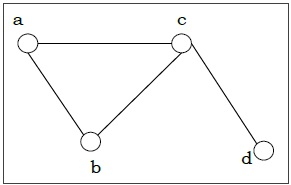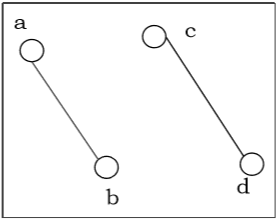# Connected vs Disconnected Graphs

## Connected Graph

A graph is connected if any two vertices of the graph are connected by a path.Vertex 1Vertex 2PATH
aba b
aca b c, a c
ada b c d, a c d
bcb a c , b c
cdc d

## Disconnected Graph

A graph is disconnected if at least two vertices of the graph are not connected by a path. If a graph G is disconnected, then every maximal connected subgraph of G is called a connected component of the graph G.Vertex 1Vertex 2PATH
aba b
acNot Available